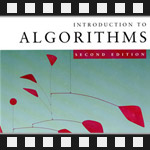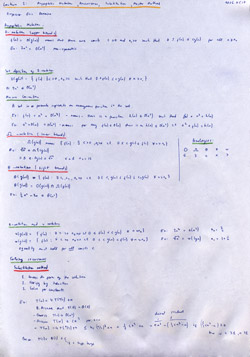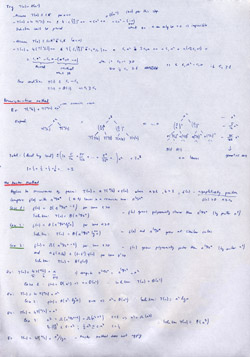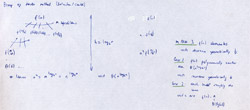I just finished watching the last lecture of MIT's "Introduction to Algorithms" course. Having a great passion for all aspects of computing, I decided to share everything I learned with you. This is the first post in an article series about this course.

As I wrote earlier, I am very serious about watching video lectures. If they are math-intensive, I usually take notes as if I were in the classroom. Lectures in this course were exactly like that -- logarithms, big-o's, thetas, expectations, and all the other math guys fighting with each other on the blackboards.

There are totally 23 video lectures, each around 1 hour 20 minutes long. I will be posting about 2 - 3 lectures at a time which will result in approximately 10 blog posts. Each post will contain annotated lecture, along with embedded video of the lecture and a time-stamped list of topics covered in the lecture. I will also post the notes I took myself as I watched the lectures. Actually, I just bought a Canon CanonScan 4400F scanner just for this purpose.

Understanding and designing effective algorithms is a very important skill for a top-notch programmer. You can still do good without knowing much about algorithms, but knowing them makes you superior. There are two kinds of people, those who can design effective algorithms and those who don't.

## Lecture 1: Analysis of Algorithms

The first lecture is given by the famous professor Charles E. Leiserson. He is the author of a popular book on algorithms.

He starts the lecture by explaining what this course and algorithms will be all about. He says that this course will be about "Analysis of Algorithms" and states: "Analysis of algorithms is the theoretical study of computer program performance and resource usage".

Designing great software is not just about performance. Charles presents a list of 12 things that can be more important than performance. Just for comparison with algorithms guru, what do you think can be more important than performance?

Here is the list of things more important than performance that Charles presented:

• modularity,
• correctness,
• maintainability,
• security,
• functionality,
• robustness,
• user-friendliness,
• programmer's time,
• simplicity,
• extensibility,
• reliability, and
• scalability.

He also asks "Why study algorithms and performance at all?". He and students answer:

• Sometimes performance is correlated with user-friendliness.
• Performance draws line between feasible and unfeasible.
• Algorithms give language for talking about program behavior.
• Performance can be used to "pay" for other things, such as security, features and user-friendliness.

The lecture continues with the definition of the Sorting Problem - given a sequence (a1, a2, ..., an) of numbers, permute them in such a way that a1 <= a2 <= ... <= an.

There are various algorithms to solve this problem. Two algorithms are presented to solve this problems - one of them is Insertion Sort and the other is Merge Sort.

Running time of these algorithms is analyzed by introducing Asymptotic Analysis and Recursion Trees.

Here is the whole lecture:

Topics covered in lecture 1:

• [17:15] Main topic of the course - Analysis of algorithms.
• [19:00] What's more important than performance?
• [22:03] Why study algorithms and performance?
• [27:45] The sorting problem.
• [29:30] Insertion sort algorithm
• [34:30] Example of Insertion sort.
• [36:25] Running time of algorithms.
• [39:39] Definition of worst-case, average-case and best-case types of analysis.
• [46:50] How to analyze the Insertion sort's worst-case running time?
• [49:28] BIG IDEA - Asymptotic analysis.
• [50:49] Asymptotic notation - theta notation.
• [57:14] Insertion sort analysis.
• [01:02:42] Is Insertion sort fast?
• [01:03:40] Merge sort algorithm.
• [01:05:25] Example of Merge subroutine of Merge sort.
• [01:08:15] Analysis of Merge sort's running time.
• [01:10:55] Recurrence equation for Merge sort.
• [01:13:15] Recursion tree solution of the Merge sort's recurrence equation.

Lecture 1 notes:

## Lecture 2: Asymptotic Notation

Lecture 2, on the other hand, is given by the youngest professor in the history of MIT – Erik Demaine. He became professor at MIT at 20.

This lecture is all about mathematical notation (Asymptotic Notation) used in the analysis of algorithms. It's the big-o notation, big omega notation, theta notation, small-o and small-omega notation.

The second half of the lecture is devoted to solving recurrence equations. Three methods are presented:

• Substitution method
• Recursion-tree method
• The Master method

Here is the whole lecture:

Topics covered in lecture 2:

• [01:25] Big-o (upper bounds) notation.
• [03:58] Set definition of big-o notation.
• [05:25] The meaning of O(h(n)) in notation f(n) = g(n) + O(h(n)).
• [10:20] Big-omega (lower bounds) notation.
• [11:40] Analogies of O, ? and ? to comparison operations of real numbers.
• [12:28] Theta (tight bounds) notation.
• [13:40] Small-o and small-omega notation.
• [17:03] Solving recurrences: substitution method.
• [37:56] Recursion-tree method.
• [49:00] The Master method.
• [01:02:00] Proof sketch of the Master method.

Lecture 2 notes:Lecture 2, page 1 of 2.Lecture 2, page 2 of 2.Lecture 2. Sketch of Master's theorem proof.

Have fun absorbing all this information! Until next post.